# Transformations

Standard

##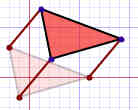Slide!##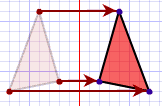Flip!##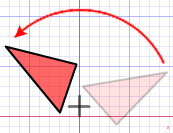Turn Around!After any of these transformations (turn around, flip or slide), the shape still has the same size, area, angles and line lengths.  In all these transformations congruence is preserved.

## Congruent or Similar

If one shape can become another using only Turns, Flips and/or Slides, then the two shapes are called Congruent.

Two shapes are Similar if you need to Resize for one shape to become another (you may also Turn, Flip and/or Slide).

## Dilation

The other important Transformation is Dilation (also called reduction or enlargement). The new image becomes bigger or smaller.

## DilationEnlarge or ReduceSo, if one shape can become another using transformation, the two shapes are either Congruent or  Similar.

If one shape is transformed… The image is ____ to the original.
… using only Rotate, Reflect and/or Translate### Congruent

… using a Dilation (may also Rotate, Reflect
and/or Translate)# Proportionality

Standard

### Proportionality

When data is PROPORTIONAL it:

-Has to start at the Origin (0,0). Y-Intercept is 0.
-Has a constant rate of change (m=y/x)
-Is Linear
-Has an equation in the form of y=mxWhen data is NON-PROPORTIONAL it is missing one of the following:
-Does not start at the Origin (0,0). Y-Intercept is not 0.
-Does not have a constant rate of change (m=y/x)
-Linear
-Equation is in the form of y=mx+b# Functions

Standard

## A Function is Special

A function is a type of equation.

A function has special rules it must follow:

In a function there can ONLY be ONE output(y) for every input (x).

Just like I stated before, a Function is Special! 🙂

• It must work for every possible input value
• And it has only one relationship for each input value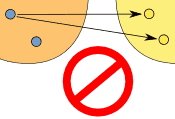THIS IS A FUNCTION!                  THIS IS NOT A FUNCTION!

### Example: y = x²

FUNCTIONCould also be written as a table:

X: x Y: x2
3 9
1 1
0 0
4 16
-4 16

It is a function, because:

• Every element in X is related to Y
• No element in X has two or more relationships
• The X values DO NOT repeat

So it follows the rules.

(Notice how both 4 and -4 relate to 16, which is allowed since 4 and -4 are two different numbers.)

### Example: This relationship is not a function:

NOT A FUNCTIONThis is not a function, for these reasons:

• Value “3” in X has no Y
• Value “4” in X has no Y
• Value “5” is related to more than one value in Y
• REMEMBER THE X VALUE CANNOT REPEAT

# Unit Rate

Standard

Unit rates are about the amount for 1 unit.

We use Ratio Tables to help solve for rates.
Our Ratio table looks like a tic-tac-toe table.We MUST label our table to know where to put our information

Ex. Bob drives 100 miles in 5 hours. How many miles does he travel every hour?Now we fill in the information that is given.

Bob drives 100 miles in 5 hours. How many miles does he travel every hour?Once we have entered what was given we need to see what the question is asking us.

Ex. Bob drives 100 miles in 5 hours. How many miles does he travel every hour?

Ask yourself, is the problem asking how many hours it takes for 1 mile or how many miles for 1 hour???????

If you said how many miles for 1 hour, you are correct!

So now we enter that into our Ratio Table.The x is the unknown. This is what we are solving for.To solve for x, we multiply 100 and 1. Then divide by the number left over which is 5.

We end up with x = 20.

So Bob drives 20 miles per hour.

Click here to watch a video with a different way to find unit rate.

Test yourself on unit rates here!

# Slope Intercept Form

Standard

### y = mx + b

m = Slope (how steep the line is)

b = the Y Intercept (where the line crosses the Y axis)\### How do you find “m” and “b“?

• b is easy: just see where the line crosses the Y axis.
• m (the Slope) needs some calculation. Remember we like to write slope like a fraction.

## Example 1)The fastest and easiest thing to find first when looking at a graph is the y-intercept (b).

Here we see that the line crosses the y-axis at positive 1.

So, b = 1 .

Now to find the slope (m) we will use rise over run:

• Rise is how far up
• Run is how far along

In this example the rise is 2 and the run is 1.

So, m = 2/1 .

Now that I know m = 2/1 and b = 1 I can plug them into the equation for slope intercept form y = mx + b.

y = (2/1) x + 1

^^^^^ This is the equation of the line.

# Slope

Standard
Slope is about how steep a line is.
When finding the slope from a line on a graph we use the method of rise over run .
• Rise is how far up
• Run is how far alongYou can also think of it as the change in y over the change in x.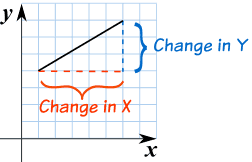EXAMPLE #1:The slope here is 4/6 which can simplify to 2/3.
EXAMPLE #2:In this example the slope is 3/5.

# Practice Problems

TEST YOUR SELF ON LINES HERE!!!!

# Y – intercept

Standard

## Y Intercept

Where a straight line crosses the Y axis of a graph.

### Example: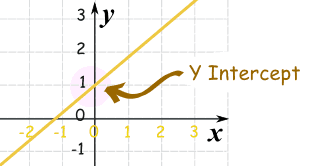In the above diagram the line crosses the Y axis at 1.

So the Y intercept is equal to 1.